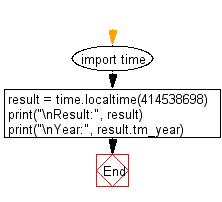﻿ Python: Print structure time in local time - w3resource# Python: Print structure time in local time

## Python Datetime: Exercise-61 with Solution

Write a Python program that takes a given number of seconds and pass since epoch as an argument. Print structure time in local time.

Sample Solution:

Python Code:

``````import time
result = time.localtime(414538698)
print("\nResult:", result)
print("\nYear:", result.tm_year)
```
```

Sample Output:

```Result: time.struct_time(tm_year=1983, tm_mon=2, tm_mday=19, tm_hour=21, tm_min=38, tm_sec=18, tm_wday=5, tm_yday=50, tm_isdst=0)

Year: 1983
```

Flowchart:## Visualize Python code execution:

The following tool visualize what the computer is doing step-by-step as it executes the said program:

Python Code Editor:

What is the difficulty level of this exercise?

Test your Python skills with w3resource's quiz

﻿

## Python: Tips of the Day

Free up Memory:

• Manual garbage collection can be performed on timely or event based mechanism.
```import gc
collected_objects = gc.collect()
```